0x02 神经网络的数学基础

2-1 神经网络所需函数

• 一次、二次函数

• 单位阶跃函数

• 指数函数和Sigmod函数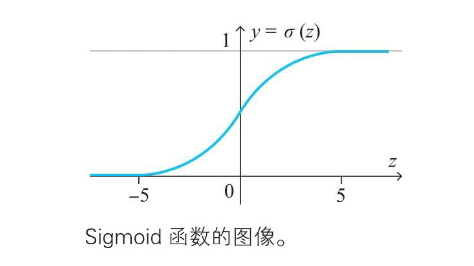• 正态分布的概率密度函数

用计算机实际确定神经网络时，必须设定权重和偏置的初始值。求初始值时，正态分布(normal distribution)是一个有用的工具。使用服从这个分布的随机数，容易取得好的结果。

2-5 矩阵基础

Hadamard 乘积：哈达玛积，即$C_{ij}=A_{ij}*B_{ij}$

2-6、7 导数基础和偏导数

Sigmod函数导数：$\sigma'(x) = \sigma(x)*(1-\sigma(x))$

2-8~12 链式法则和梯度下降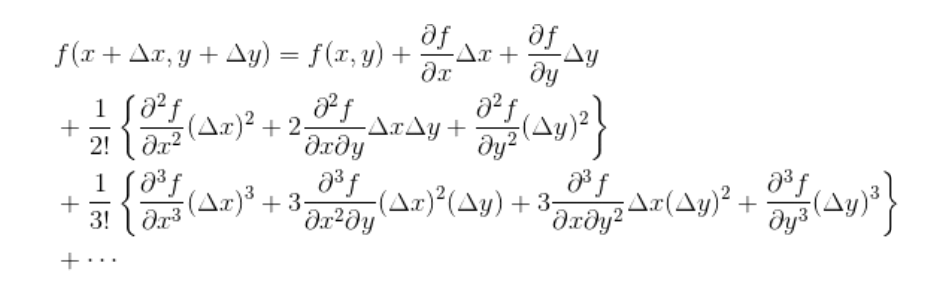$\Delta x = -\eta\nabla f$

3 最优化

3-1 参数和变量

**参数：**从数学上看，神经网络是一种用于数据分析的模型，这个模型是由权重和偏置确定的。像权重和偏置这种确定数学模型的常数称为模型的参数。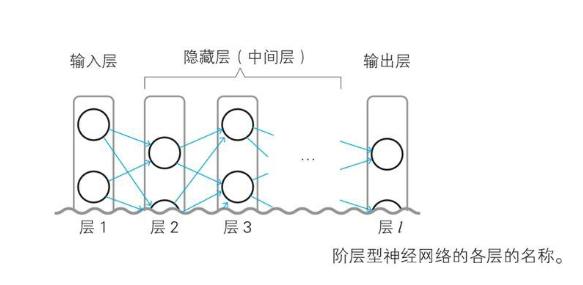$x_i$ 表示输入层(层1)的第i个神经单元的输入的变量。由于输入层的神经单元的输入和输出为同一值，所以也是表示输出的变量。此外，这个变量名也作为神经单元的名称使用
$w_{ji}^l$ 从层 l-1 的第i个神经单元指向层l的第j个神经单元的箭头的权重。请注意ij的顺序。这是神经网络的参数
$z_j^l$ 表示层l的第j个神经单元的加权输入的变量
$b_j^l$ l的第j个神经单元的偏置。这是神经网络的参数
$a_j^l$ l的第j个神经单元的输出变量。此外，这个变量名也作为神经单元的名称使用

3-2~4 变量、预测值和正解、代价函数

Pytorch常见的Loss函数：

L1Loss 计算output和target之差的绝对值 mean/sum
MSELoss 计算output和target之差的平方 mean/sum
CrossEntropyLoss 将输入经过 softmax 激活函数之后，再计算其与 target 的交叉熵损失。即该方法将 nn.LogSoftmax() 和nn.NLLLoss() 进行了结合。严格意义上的交叉熵损失函数应该是 nn.NLLLoss() 在多分类任务中，经常采用 softmax 激活函数+交叉熵损失函数，因为交叉熵描述了两个概率分布的差异，然而神经网络输出的是向量，并不是概率分布的形式。所以需要 softmax 激活函数将一个向量进行“归一化”成概率分布的形式，再采用交叉熵损失函数计算 loss。mean/sum
NLLLoss 常用于多分类任务，但是 input 在输入 NLLLoss() 之前，需要对 input 进行 log_softmax 函数激活，即将 input 转换成概率分布的形式，并且取对数。 如果不想让网络的最后一层是log_softmax层的话，就可以采用 CrossEntropyLoss 完全代替此函数。mean/sum
KLDivLoss KL散度又称为相对熵 (Relative Entropy)，用于描述两个概率分布之间的差异。 KL散度值是不对称的。mean/sum

0x04 误差反向传播

4-1 梯度下降回顾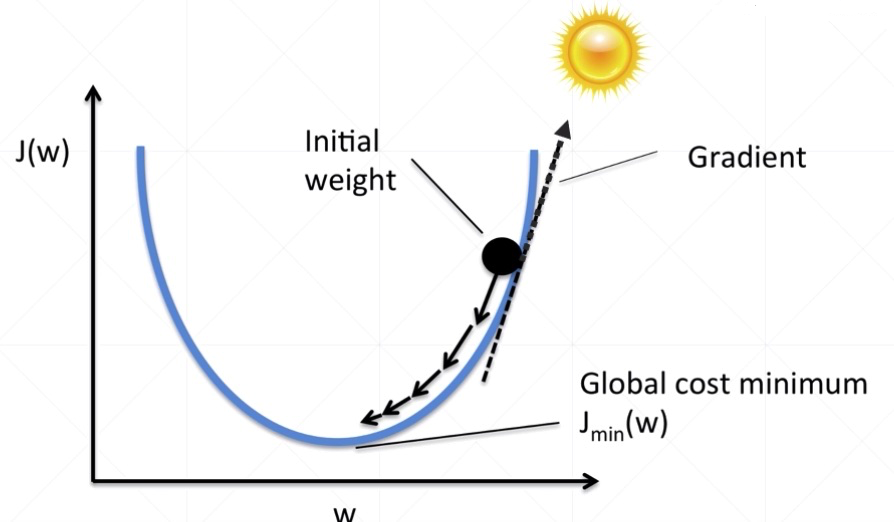4-2 神经单元误差$\delta_j^l$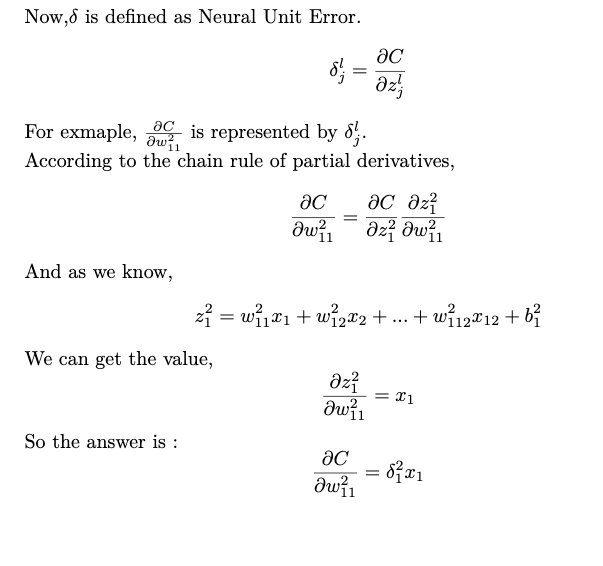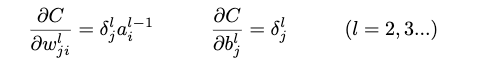4-3 误差反向传播法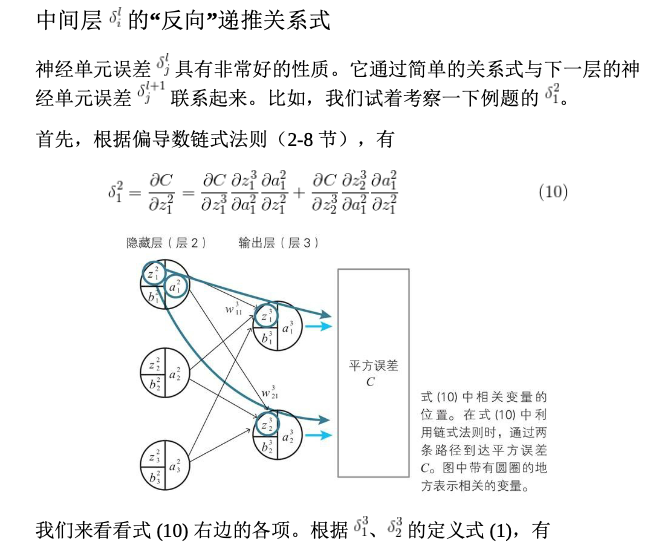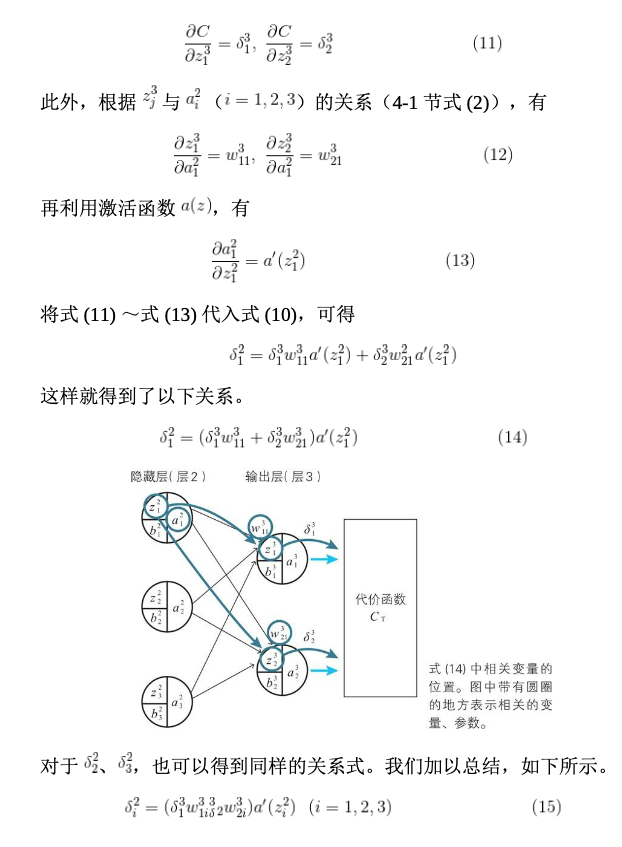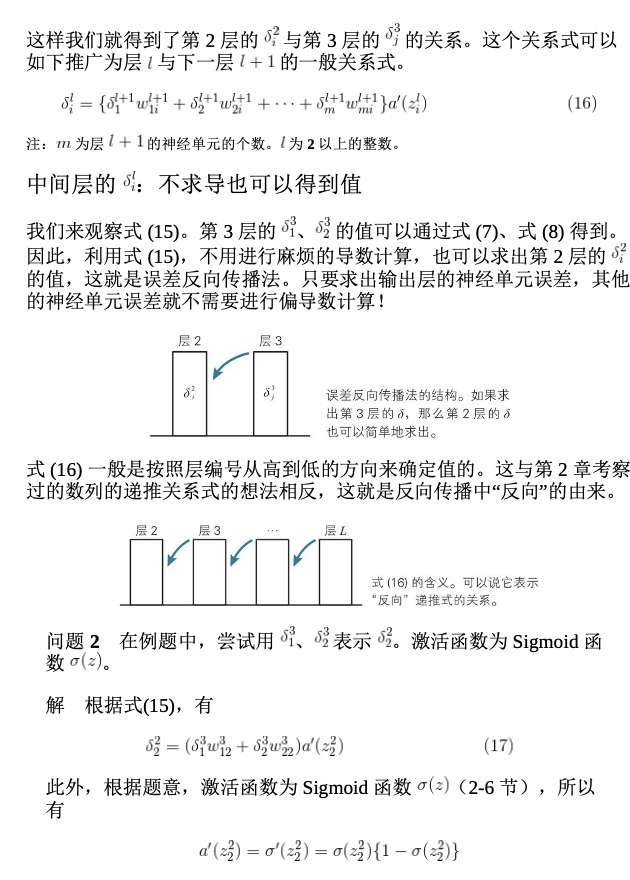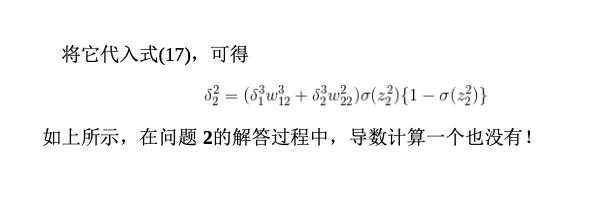0x05 卷积神经网络

L2池化 使用对象区域的平方和的平方根作为代表值